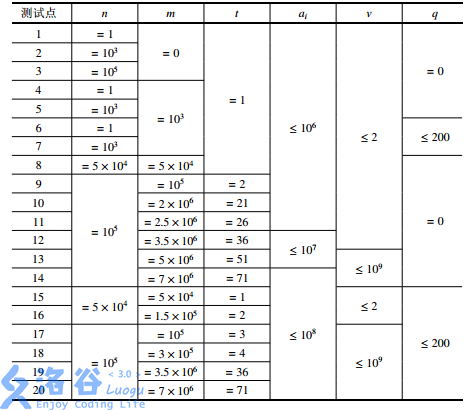# noip2016 蚯蚓

## 题目描述

(m为非负整数）

•m秒内，每一秒被切断的蚯蚓被切断前的长度（有m个数）

•m秒后，所有蚯蚓的长度（有n+m个数)。

## 输入输出样例

3 7 1 1 3 1
3 3 2

3 4 4 4 5 5 6
6 6 6 5 5 4 4 3 2 2

3 7 1 1 3 2
3 3 2

4 4 5
6 5 4 3 2

3 7 1 1 3 9
3 3 2

//空行
2

## 说明

【样例解释1】

1秒后：一只长度为3的蚯蚓被切成了两只长度分别为1和2的蚯蚓，其余蚯蚓的长度增加了1。最终4只蚯蚓的长度分别为(1,2),4,3。括号表示这个位置刚刚有一只蚯蚓被切断

2秒后：一只长度为4的蚯蚓被切成了1和3。5只蚯蚓的长度分别为：2,3,(1,3),4。

3秒后：一只长度为4的蚯蚓被切断。6只蚯蚓的长度分别为：3,4,2,4,(1,3)。

4秒后：一只长度为4的蚯蚓被切断。7只蚯蚓的长度分别为：4,(1,3),3,5,2,4。

5秒后：一只长度为5的蚯蚓被切断。8只蚯蚓的长度分别为：5,2,4,4,(1,4),3,5。

6秒后：一只长度为5的蚯蚓被切断。9只蚯蚓的长度分别为：（1,4),3,5,5,2,5,4,6。

7秒后：一只长度为6的蚯蚓被切断。10只蚯蚓的长度分别为：2,5,4,6,6,3,6,5,(2,4)。所以，7秒内被切断的蚯蚓的长度依次为3,4,4,4,5,5,6。7秒后，所有蚯蚓长度从大到小排序为6,6,6,5,5,4,4,3,2,2

【样例解释2】

【样例解释3】

【数据范围】要想做出这道题，就必须要跳出优先队列的思维。一个常用的优化方法就是手写堆，不过效率不高，如果能够手写队列就好了.其实如果我们把原序列按照降序排序，每次砍掉最长的蚯蚓，更长的一半放在一个队列里，另一半放在另一个队列里，再套上之前所说的优化，就构成了3个单调队列，为什么会构成3个单调队列呢？因为我们是先砍长的蚯蚓，而且是等比例砍的，按照之前的优化，每个被砍的部分只会被减一次q,所以是3个单调队列，那么我们每次只需要取3个队列中的队首的最大值来砍即可.

#include <cstdio>
#include <cstring>
#include <iostream>
#include <algorithm>
#include <queue>
#include <stack>
#include <cstdio>
#include <iostream>
#include <cstring>
#include <algorithm>
#include <string>

using namespace std;

int n, m, q, u, v, t,a,cnt,tot1 = 1,tot2 = 1,tot3 = 1,b,c,tail2,tail3,sum;
double p;

bool cmp(int a, int b)
{
return a > b;
}int getmax()
{
int temp = -1000000000;
bool flag1 = 0, flag2 = 0, flag3 = 0;
if (tot1 > n)
flag1 = 1;
if (tot2 > tail2)
flag2 = 1;
if (tot3 > tail3)
flag3 = 1;
if (flag1 && flag2)
{
cnt = 3;
return c[tot3];
}
if (flag1 && flag3)
{
cnt = 2;
return b[tot2];
}
if (flag2 && flag3)
{
cnt = 1;
return a[tot1];
}
if (flag1)
{
if (b[tot2] > c[tot3])
{
cnt = 2;
return b[tot2];
}
else
{
cnt = 3;
return c[tot3];
}
}
if (flag2)
{
if (a[tot1] > c[tot3])
{
cnt = 1;
return a[tot1];
}
else
{
cnt = 3;
return c[tot3];
}
}
if (flag3)
{
if (a[tot1] > b[tot2])
{
cnt = 1;
return a[tot1];
}
else
{
cnt = 2;
return b[tot2];
}
}
temp = max(a[tot1], max(b[tot2], c[tot3]));
if (temp == a[tot1])
{
cnt = 1;
return temp;
}
if (temp == b[tot2])
{
cnt = 2;
return temp;
}
if (temp == c[tot3])
{
cnt = 3;
return temp;
}
}

int main()
{
scanf("%d%d%d%d%d%d", &n, &m, &q, &u, &v, &t);
for (int i = 1; i <= n; i++)
scanf("%d", &a[i]);
sort(a + 1, a + 1 + n, cmp);
for (int i = 1; i <= m; i++)
{
int tt = getmax();
if (cnt == 1)
tot1++;
if (cnt == 2)
tot2++;
if (cnt == 3)
tot3++;
if (i % t == 0)
printf("%d ", tt + sum);
b[++tail2] = (long long)(tt + sum) * u / v - sum - q);
c[++tail3] = ((tt + sum) - (long long)(tt + sum) * u / v - sum - q);
sum += q;
}
printf("\n");
int time = 0;
while (tot1 <= n || tot2 <= tail2 || tot3 <= tail3)
{
time++;
int tt = getmax();
if (cnt == 1)
tot1++;
if (cnt == 2)
tot2++;
if (cnt == 3)
tot3++;
if (time % t == 0)
{
printf("%d ", tt + sum);
}
}

return 0;
}

posted @ 2017-07-28 23:40 zbtrs 阅读(...) 评论(...) 编辑 收藏